Monday, August 2, 2010

Here's the official write up for the DEFCON 18 Badge.

I took a little bit of time to solve 2 of the puzzles.

Puzzle #1: QR Code

One of the puzzles was a hidden QR code that looked like this: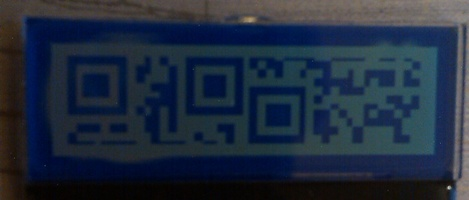Which translated to this: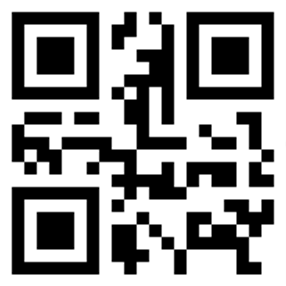Which, when scanned with a QR code reader, displayed:

```VANDALS WANG
```

If you ran into Vandal (one of the goons) at the conference, he was wearing a QR code around his neck. When scanned, it displayed:

```Vandal
Official Defcon Scavenger Hunt Judge
```

Puzzle #2: Ninja Party

Solving the key was irrelevant to the "Ninja Party" as it is known by Con goers, but it was a challenge, so me and a fellow "ninja in training" did it anyway. The first screen of the challenge looked like this: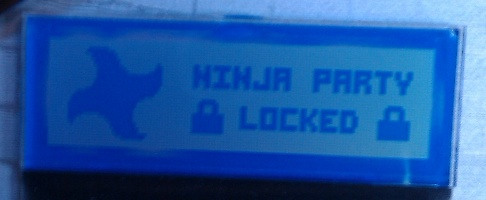When you entered the challenge, you were required to configure 15 tumblers as if you were picking a lock.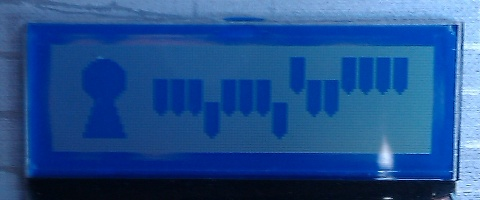If you entered the pattern correctly, the screen displayed this: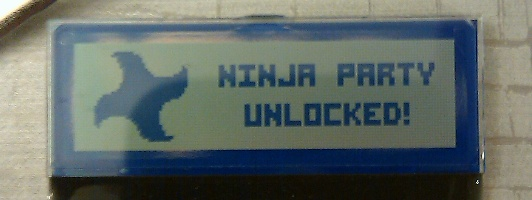We weren't about to navigate the clumsy interface and attempt to brute force the key to solve the puzzle, so we went straight to the code (C for those interested). Inside the code we found this:

```/**************************************************************/
/* NINJA ROUTINES
/**************************************************************/

int dc18_ninja_validate(uint32_t val)
{
uint16_t a, b;

a = (uint16_t)(val & 0xfff);
b = (uint16_t)(val >> 12);

if((a ^ b) == 0x916)
{
return 1;
}
return 0;
}

// encode tumbler states into 24-bit value
uint32_t dc18_encode_tumblers(tumbler_state_type *tumblers)
{
uint32_t x = 0, j = 1;
uint16_t i;

for(i = 0; i < TUMBLERS_PER_IMAGE; i++)
{
x += tumblers[i] * j;
j *= 3;
}

return x;
}
```

After some reverse engineering on paper, we solved the encoding and validation algorithms and wrote the following Python script to display every possible key. With 3^15 possibilities, there were only 3,503 valid codes. The script creates a file with all of the possible keys in it.

```def base10toN(num,n):
"""Change a  to a base-n number.
Up to base-36 is supported without special notation."""
num_rep={10:'a',
11:'b',
12:'c',
13:'d',
14:'e',
15:'f',
16:'g',
17:'h',
18:'i',
19:'j',
20:'k',
21:'l',
22:'m',
23:'n',
24:'o',
25:'p',
26:'q',
27:'r',
28:'s',
29:'t',
30:'u',
31:'v',
32:'w',
33:'x',
34:'y',
35:'z'}
new_num_string=''
current=num
while current!=0:
remainder=current%n
if 36>remainder>9:
remainder_string=num_rep[remainder]
elif remainder>=36:
remainder_string='('+str(remainder)+')'
else:
remainder_string=str(remainder)
new_num_string=remainder_string+new_num_string
current=current/n
return new_num_string ## end of http://code.activestate.com/recipes/65212/ }}}

file = open('keys', 'w')
#14348907
n = 0
cnt = 0
while n <= 14348907:
a = n & 4095
#print a
b = n >> 12
#print b
if a ^ b == 2326:
cnt = cnt + 1
print str(cnt) + ":" + str(n) + ":" + str(base10toN(n, 3).rjust(15, '0'))[::-1]
file.writelines(str(cnt) + ":" + str(n) + ":" + str(base10toN(n, 3).rjust(15, '0'))[::-1] + "\n")
n += 1
file.closed
```

Like what you see? Join me for live training! See the Training page for more information.